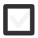# Draw a COORDINATE SYSTEM with axes & y = 3x + 1. What is the rate of change of the line? Where is the origin?158 views
In 7-9
edited

## 2 lessons

+1 vote
by Guru (6.1k)
edited

Graphing equations

Math is often ambiguous at best, especially when things get more and more complicated. However, this is also true for real life: How do you make sense of team or player statistics in sports, for example? How do the meteorologists figure out where the weather patterns are taking us? The common denominator is graphing. With visualisations we can make complicated things make sense much easier.

Let’s learn the theory:

Exercises:

1. Look at the graph. What is…
• The largest value of the function?
• Smallest value of the function?
• Which value of x gives the the largest value?
• What is the value of the finction when x = -3?2. Graph the following equations using intercepts.

• Graph 2x + 4 = 0
• Graph 2y - 6 = 0

3. Graph the following three equations on the same set of coordinate axes.

• y=x+1y=2x+1y=−x+1

4. Graph the following three equations on the same set of coordinate axes.

• y=2x+1y=2xy=2x−1

Credit: math.libretexts.org

Great lesson!